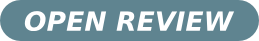# Topological quantum phase transition in strongly correlated Kondo insulators in 1D

• Franco Thomas Lisandrini Instituto de Física Rosario (CONICET) and Facultad de Ciencias Exactas, Ingeniería y Agrimensura, Universidad Nacional de Rosario.
• Alejandro Martín Lobos Instituto de Física Rosario (CONICET), Argentina.
• Ariel Oscar Dobry Instituto de Física Rosario (CONICET) and Facultad de Ciencias Exactas, Ingeniería y Agrimensura, Universidad Nacional de Rosario
• Claudio Javier Gazza Instituto de Física Rosario (CONICET) and Facultad de Ciencias Exactas, Ingeniería y Agrimensura, Universidad Nacional de Rosario
Keywords: Topological Insulators, Topological Quantum Phase, Entanglement Entropy, Entanglement Spectrum

### Abstract

We investigate, by means of a field-theory analysis combined with the density-matrix renormalization group (DMRG) method, a theoretical model for a strongly
correlated quantum system in one dimension realizing a topologically-ordered  Haldane phase ground state. The model consists of a spin-1/2 Heisenberg chain coupled to a tight-binding chain via two competing Kondo exchange couplings of different type: a $s$-wave'' Kondo coupling ($J^s_{K}$), and a less common $p$-wave'' ($J^p_{K}$) Kondo coupling. While the first coupling is the standard Kondo interaction studied in many condensed-matter systems, the latter has been recently introduced by Alexandrov and Coleman [Phys. Rev. B 90, 115147 (2014)] as a possible mechanism leading to a topological Kondo-insulating ground state in one dimension. As a result of this competition, a topological quantum phase transition (TQPT) occurs in the system for a critical value of the ratio $J^s_{K}/J^p_{K}$, separating a (Haldane-type) topological phase  from a topologically trivial ground state where the system can be essentially described as a product of local singlets. We study and characterize the TQPT by means of  the magnetization profile, the entanglement entropy and the full entanglement spectrum of the ground state. Our results might be relevant to understand how topologically-ordered phases of fermions emerge in strongly interacting quantum systems.

Received: 1 July 2016,  Accepted: 30 August 2016; Edited by: K.  Hallberg;  Reviewed by: D. C. Cabra, Instituto de Física La Plata, La Plata, Argentina; DOI: http://dx.doi.org/10.4279/PIP.080005

Cite as: F T Lisandrini, A M Lobos, A O Dobry, C J Gazza, Papers in Physics 8, 080005 (2016)